x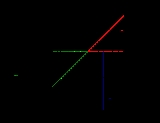Trajectory of a projectileOverview

In physics
Physics
Physics is a natural science that involves the study of matter and its motion through spacetime, along with related concepts such as energy and force. More broadly, it is the general analysis of nature, conducted in order to understand how the universe behaves.Physics is one of the oldest academic...

, the ballistic trajectory of a projectile is the path that a thrown or launched projectile
Projectile
A projectile is any object projected into space by the exertion of a force. Although a thrown baseball is technically a projectile too, the term more commonly refers to a weapon....

will take under the action of gravity, neglecting all other forces, such as friction
Friction
Friction is the force resisting the relative motion of solid surfaces, fluid layers, and/or material elements sliding against each other. There are several types of friction:...

from air resistance, without propulsion
Vehicle propulsion
Vehicle propulsion refers to the act of moving an artificial carrier of people or goods over any distance. The power plant used to drive the vehicles can vary widely. Originally, humans or animals would have provided the propulsion system, later being supplemented by wind power...

.

The United States Department of Defense
United States Department of Defense
The United States Department of Defense is the U.S...

and NATO define a ballistic
Ballistics
Ballistics is the science of mechanics that deals with the flight, behavior, and effects of projectiles, especially bullets, gravity bombs, rockets, or the like; the science or art of designing and accelerating projectiles so as to achieve a desired performance.A ballistic body is a body which is...

trajectory
Trajectory
A trajectory is the path that a moving object follows through space as a function of time. The object might be a projectile or a satellite, for example. It thus includes the meaning of orbit—the path of a planet, an asteroid or a comet as it travels around a central mass...

as a trajectory traced after the propulsive force is terminated and the body is acted upon only by gravity and aerodynamic drag.

The following applies for ranges which are small compared to the size of the Earth.Encyclopedia
In physics
Physics
Physics is a natural science that involves the study of matter and its motion through spacetime, along with related concepts such as energy and force. More broadly, it is the general analysis of nature, conducted in order to understand how the universe behaves.Physics is one of the oldest academic...

, the ballistic trajectory of a projectile is the path that a thrown or launched projectile
Projectile
A projectile is any object projected into space by the exertion of a force. Although a thrown baseball is technically a projectile too, the term more commonly refers to a weapon....

will take under the action of gravity, neglecting all other forces, such as friction
Friction
Friction is the force resisting the relative motion of solid surfaces, fluid layers, and/or material elements sliding against each other. There are several types of friction:...

from air resistance, without propulsion
Vehicle propulsion
Vehicle propulsion refers to the act of moving an artificial carrier of people or goods over any distance. The power plant used to drive the vehicles can vary widely. Originally, humans or animals would have provided the propulsion system, later being supplemented by wind power...

.

The United States Department of Defense
United States Department of Defense
The United States Department of Defense is the U.S...

and NATO define a ballistic
Ballistics
Ballistics is the science of mechanics that deals with the flight, behavior, and effects of projectiles, especially bullets, gravity bombs, rockets, or the like; the science or art of designing and accelerating projectiles so as to achieve a desired performance.A ballistic body is a body which is...

trajectory
Trajectory
A trajectory is the path that a moving object follows through space as a function of time. The object might be a projectile or a satellite, for example. It thus includes the meaning of orbit—the path of a planet, an asteroid or a comet as it travels around a central mass...

as a trajectory traced after the propulsive force is terminated and the body is acted upon only by gravity and aerodynamic drag.

The following applies for ranges which are small compared to the size of the Earth. For longer ranges see sub-orbital spaceflight
Sub-orbital spaceflight
A sub-orbital space flight is a spaceflight in which the spacecraft reaches space, but its trajectory intersects the atmosphere or surface of the gravitating body from which it was launched, so that it does not complete one orbital revolution....

.

## Notation

In the equations on this page, the following variables will be used:
• g: the gravitational acceleration
Gravitational acceleration
In physics, gravitational acceleration is the acceleration on an object caused by gravity. Neglecting friction such as air resistance, all small bodies accelerate in a gravitational field at the same rate relative to the center of mass....

—usually taken to be 9.81 m/s2 near the Earth's surface
• θ: the angle at which the projectile is launched
• v: the velocity at which the projectile is launched
• y0: the initial height of the projectile
• d: the total horizontal distance traveled by the projectile

Ballistics (gr. βάλλειν ('ba'llein'), "throw") is the science of mechanics that deals with the flight, behavior, and effects of projectiles, especially bullets, gravity bombs, rockets, or the like; the science or art of designing and accelerating projectiles so as to achieve a desired performance.
A ballistic body is a body which is free to move, behave, and be modified in appearance, contour, or texture by ambient conditions, substances, or forces, as by the pressure of gases in a gun, by rifling in a barrel, by gravity, by temperature, or by air particles. A ballistic missile is a missile only guided during the relatively brief initial powered phase of flight, whose course is subsequently governed by the laws of classical mechanics.

### Distance travelled

The total horizontal distance (d) travelled.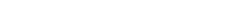When the surface the object is launched from and is flying over is flat (the initial height is zero), the distance travelled is: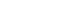Thus the maximum distance is obtained if θ is 45 degrees. This distance is: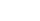For explicit derivations of these results, see Range of a projectile
Range of a projectile
right|thumb|250 px|The path of this projectile launched from a height y0 has a range d.In physics, assuming a flat Earth with a uniform gravity field, a projectile launched with specific initial conditions will have a predictable range. As in Trajectory of a projectile, we will use:The following...

.

### Time of flight

The time of flight (t) is the time it takes for the projectile to finish its trajectory.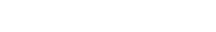As above, this expression can be reduced to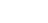if θ is 45° and y0 is 0.

The above results are found in Range of a projectile
Range of a projectile
right|thumb|250 px|The path of this projectile launched from a height y0 has a range d.In physics, assuming a flat Earth with a uniform gravity field, a projectile launched with specific initial conditions will have a predictable range. As in Trajectory of a projectile, we will use:The following...

.

### Angle of reach

The "angle of reach" (not quite a scientific term) is the angle (θ) at which a projectile must be launched in order to go a distance d, given the initial velocity v.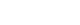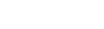### Height at x

The height y of the projectile at distance x is given by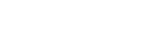.

The third term is the deviation from traveling in a straight line.

### Velocity at x

The magnitude,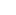of the velocity of the projectile at distance x is given by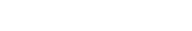.

#### Derivation

The magnitude |v| of the velocity is given by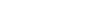,

where Vx and Vy are the instantaneous velocities in the x- and y-directions, respectively.

Here the x-velocity remains constant; it is always equal to v cos θ.

The y-velocity can be found using the formula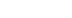by setting vi = v sin θ, a = -g, and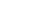. (The latter is found by taking x = (v cos θ) t and solving for t.) Then,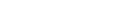and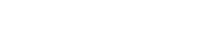.

The formula above is found by simplifying.

## Angle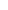required to hit coordinate (x,y)

To hit a target at range x and altitude y when fired from (0,0) and with initial velocity v the required angle(s) of launchare: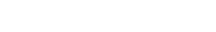Each root of the equation corresponds to the two possible launch angles so long as both roots aren't imaginary, in which case the initial velocity is not great enough to reach the point (x,y) you have selected. The greatest feature of this formula is that it allows you to find the angle of launch needed without the restriction of y = 0.

Derivation

First, two elementary formulae are called upon relating to projectile motion: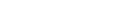(1)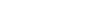(2)

Solving (1) for t and substituting this expression in (2) gives: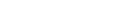(2a)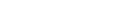(2b) (Trigonometric identity)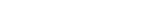(2c) (Trigonometric identity)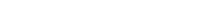(2d) (Algebra)

Let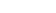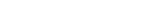(2e) (Substitution)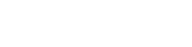(2f) (Quadratic formula)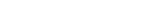(2f) (Algebra)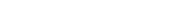(2g) (Substitution)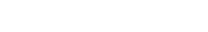(2h) (Algebra)

Also, if instead of a coordinate (x,y) you're interested in hitting a target at distance r and angle of elevation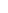(polar coordinates), use the relationships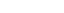and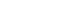and substitute to get: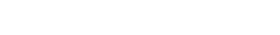## Trajectory of a projectile with air resistance

Air resistance will be taken to be in direct proportion to the velocity of the particle (i.e.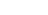). This is valid at low speed (low Reynolds number), and this is done so that the equations describing the particle's motion are easily solved. At higher speed (high Reynolds number) the force of air resistance is proportional to the square of the particle's velocity (see drag equation
Drag equation
In fluid dynamics, the drag equation is a practical formula used to calculate the force of drag experienced by an object due to movement through a fully enclosing fluid....

). Here,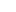,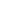and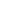will be used to denote the initial velocity, the velocity along the direction of x and the velocity along the direction of y, respectively. The mass of the projectile will be denoted by m. For the derivation only the case where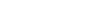is considered. Again, the projectile is fired from the origin (0,0).

For this assumption, that air resistance may be taken to be in direct proportion to the velocity of the particle is not correct for a typical projectile in air with a velocity above a few tens of meters/second, and so this equation should not be applied to that situation.
The free body diagram
Free body diagram
A free body diagram, also called a force diagram, is a pictorial representation often used by physicists and engineers to analyze the forces acting on a body of interest. A free body diagram shows all forces of all types acting on this body. Drawing such a diagram can aid in solving for the unknown...

on the right is for a projectile that experiences air resistance and the effects of gravity. Here, air resistance is assumed to be in the direction opposite of the projectile's velocity.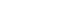is written due to the initial assumption of direct proportionality implies that the air resistance and the velocity differ only by a constant arbitrary factor with units of N*s/m.

As an example, say that when the velocity of the projectile is 4 m/s, the air resistance is 7 newtons (N). When the velocity is doubled to 8 m/s, the air resistance doubles to 14 N accordingly. In this case, k = 7/4 N x s/m. Note that k is needed in order to relate the air resistance and the velocity by an equal sign: otherwise, it would be stating incorrectly that the two are always equal in value (i.e. 1 m/s of velocity gives 1 N of force, 2 m/s gives 2 N etc.) which isn't always the case, and also it keeps the equation dimensionally correct (a force and a velocity cannot be equal to each other, e.g. m/s = N). As another quick example, Hooke's Law
Hooke's law
In mechanics, and physics, Hooke's law of elasticity is an approximation that states that the extension of a spring is in direct proportion with the load applied to it. Many materials obey this law as long as the load does not exceed the material's elastic limit. Materials for which Hooke's law...

(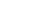) describes the force produced by a spring when stretched a distance x from its resting position, and is another example of a direct proportion: k in this case has units N/m (in metric).

To show why k = 7/4 N·s/m above, first equate 4 m/s and 7 N: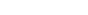(Incorrect)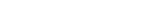(Introduction of k)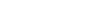(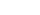cancels)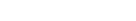For more on proportionality, see: Proportionality (mathematics)
Proportionality (mathematics)
In mathematics, two variable quantities are proportional if one of them is always the product of the other and a constant quantity, called the coefficient of proportionality or proportionality constant. In other words, are proportional if the ratio \tfrac yx is constant. We also say that one...

The relationships that represent the motion of the particle are derived by Newton's Second Law, both in the x and y directions.
In the x direction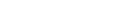and in the y direction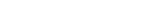.

This implies that: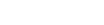(1),

and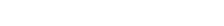(2)

Solving (1) is an elementary differential equation
Differential equation
A differential equation is a mathematical equation for an unknown function of one or several variables that relates the values of the function itself and its derivatives of various orders...

, thus the steps leading to a unique solution forand, subsequently,will not be enumerated. Given the initial conditions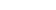(where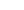is understood to be the x component of the initial velocity) and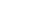for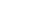: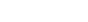(1a)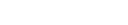(1b)

While (1) is solved much in the same way, (2) is of distinct interest because of its non-homogeneous nature. Hence, we will be extensively solving (2). Note that in this case the initial conditions are used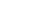and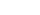when.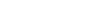(2)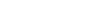(2a)

This first order, linear, non-homogeneous differential equation may be solved a number of ways, however, in this instance it will be quicker to approach the solution via an integrating factor
Integrating factor
In mathematics, an integrating factor is a function that is chosen to facilitate the solving of a given equation involving differentials. It is commonly used to solve ordinary differential equations, but is also used within multivariable calculus, in this case often multiplying through by an...

: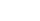.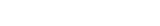(2c)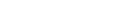(2d)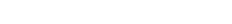(2e)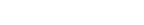(2f)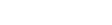(2g)

And by integration we find: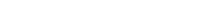(3)

Solving for our initial conditions: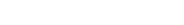(2h)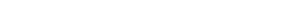(3a)

With a bit of algebra to simplify (3a):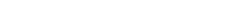(3b)

An example is given using values for the mass and terminal velocity for a baseball
Baseball
Baseball is a bat-and-ball sport played between two teams of nine players each. The aim is to score runs by hitting a thrown ball with a bat and touching a series of four bases arranged at the corners of a ninety-foot diamond...

taken from http://hyperphysics.phy-astr.gsu.edu/hbase/airfri2.html#c3.
m = 0.145 kg (5.1 oz)
v0 = 44.7 m/s (100 mph)
g = -9.81 m/s² (-32.2 ft/s²)
vt = -33.0 m/s (-73.8 mph)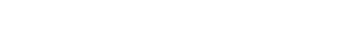.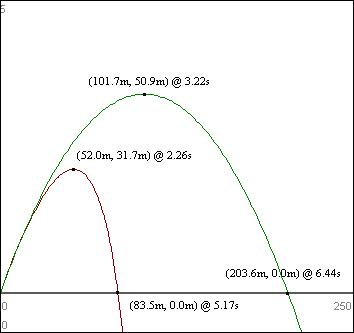]

The red path is the path taken by the projectile modeled by the equations derived above, and the green path is taken by an idealized projectile, one that ignores air resistance altogether. (3.28 ft/m) Ignoring air resistance is not ideal in this scenario, as with no air resistance, a home run could be hit with 270 ft to spare. (The mechanics of pitching at 45 degrees notwithstanding.) And in some cases it's more accurate to assume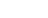, meaning when air resistance increases by a factor of p the resistance increases by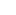. In the first example of proportionality, where the velocity was doubled to 8 m/s, the air resistance would instead be quadrupled (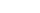) to 28 N: this only adds to the large amount of error in neglecting air resistance.

• Ballistic coefficient
Ballistic coefficient
In ballistics, the ballistic coefficient of a body is a measure of its ability to overcome air resistance in flight. It is inversely proportional to the negative acceleration—a high number indicates a low negative acceleration. BC is a function of mass, diameter, and drag coefficient...

• Range of a projectile
Range of a projectile
right|thumb|250 px|The path of this projectile launched from a height y0 has a range d.In physics, assuming a flat Earth with a uniform gravity field, a projectile launched with specific initial conditions will have a predictable range. As in Trajectory of a projectile, we will use:The following...

• Trajectory
Trajectory
A trajectory is the path that a moving object follows through space as a function of time. The object might be a projectile or a satellite, for example. It thus includes the meaning of orbit—the path of a planet, an asteroid or a comet as it travels around a central mass...

In fluid dynamics, drag (sometimes called air resistance or fluid resistance) refers to forces which act on a solid object in the direction of the relative fluid flow velocity.    Unlike other resistive forces such as dry friction, which is nearly independent of velocity, drag forces depend on velocity.
Drag forces always decrease fluid velocity relative to the solid object in the fluid's path.